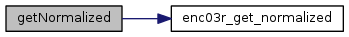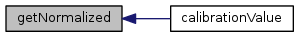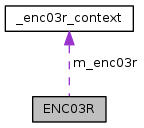upm  1.7.1 Sensor/Actuator repository for libmraa (v2.0.0)
ENC03R Class Reference

API for the ENC03R Single Axis Analog Gyro. More...

## Detailed Description

UPM module for the ENC03R single axis analog gyro. This gyroscope measures x-axis angular velocity, that is how fast the sensor is rotating around the x-axis. Calibration of the sensor is necessary for accurate readings.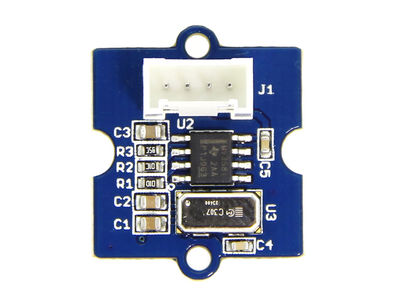// Instantiate a ENC03R on analog pin A0
upm::ENC03R gyro(0);
// The first thing we need to do is calibrate the sensor.
cout << "Please place the sensor in a stable location, and do not" << endl;
cout << "move it while calibration takes place." << endl;
cout << "This may take a couple of minutes." << endl;
gyro.calibrate(CALIBRATION_SAMPLES);
cout << "Calibration complete. Reference value: " << gyro.calibrationValue() << endl;
// Read the input and print both the raw value and the angular velocity,
// waiting 0.1 seconds between readings
while (shouldRun) {
gyro.update();
cout << "Angular velocity: " << gyro.angularVelocity() << " deg/s" << endl;
upm_delay_us(100000);
}

## Public Member Functions

ENC03R (int pin, float aref=5.0)

~ENC03R ()

void calibrate (unsigned int samples)

void update ()

float calibrationValue ()

float angularVelocity ()

void setOffset (float offset)

void setScale (float scale)

float getNormalized ()

## Protected Attributes

enc03r_context m_enc03r

## Constructor & Destructor Documentation

 ENC03R ( int pin, float aref = `5.0` )

ENC03R sensor constructor

Parameters
 pin Analog pin to use aref Reference voltage to use; default is 5.0 V

Here is the caller graph for this function: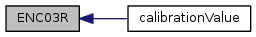~ENC03R ( )

ENC03R destructor

Here is the call graph for this function: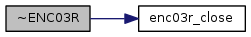## Member Function Documentation

 void calibrate ( unsigned int samples )

Calibrates the sensor by determining an analog reading over many samples with no movement of the sensor. This must be done before attempting to use the sensor.

Parameters
 samples Number of samples to use for calibration

Here is the call graph for this function: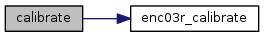void update ( void )

Update the internal state with the current reading. This function must be called prior to calling angularVelocity().

Parameters
 dev Device context

Here is the call graph for this function: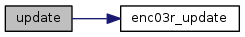float calibrationValue ( )
inline

Returns the currently stored calibration value

Returns
Current calibration value

Here is the call graph for this function: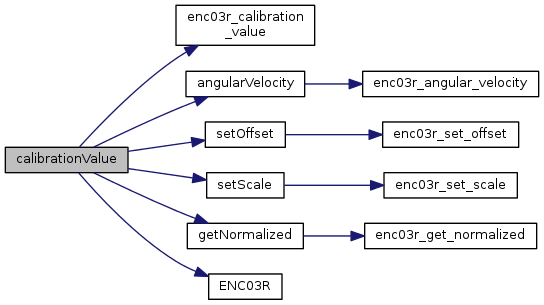float angularVelocity ( )

Computes angular velocity based on the value and stored calibration reference.

Parameters
 val Value to use to compute angular velocity
Returns
Computed angular velocity

Here is the call graph for this function: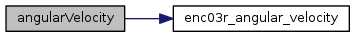Here is the caller graph for this function: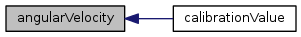void setOffset ( float offset )

Set sensor offset. The offset is applied to the return value before scaling. Default is 0.

Parameters
 offset Offset to apply to value

Here is the call graph for this function: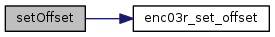Here is the caller graph for this function: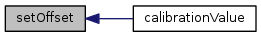void setScale ( float scale )

Set sensor scale. The return value is scaled by this value after the offset is applied. Default is 1.0.

Parameters
 scale Scale to apply to value

Here is the call graph for this function: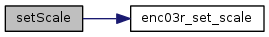Here is the caller graph for this function: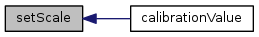float getNormalized ( )

Get a normalized ADC value from the sensor. The return value will be between 0.0 (indicating no voltage) and 1.0 indicating max voltage (aref). update() must be called prior to calling this function.

Returns